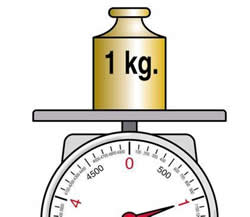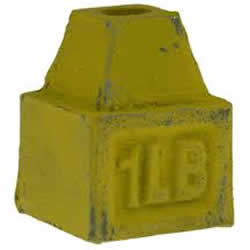# Difference between Kilo and Lbs

##### Key Difference: Kilogram is purely a unit of mass measurement. ‘lbs’ is the abbreviation and the official notion used to indicate pounds.One kilo of mass is equal to 2.2 lbs, which basically means that a kilogram (kg) is 2.2 times heavier than a pound (lbs). One kilogram is the standard unit of measurement of weight. A kilogram weighs 9.81 Newtons (N) or 2.21 pounds (lb). The kilogram is not the weight of a person,and at the same time it is to be measured as a unit of mass.‘lbs.’ is the abbreviation and the official notion used to indicate pounds. The unit of measurement is often abbreviated as ‘lb’ or ‘lbs’. According to the international convention there is only ‘lb’ and it does not have a plural form (i.e. ‘lbs’), though it has been gaining popularity in usage. The unit and its abbreviation originate from the Roman libra, while the

name pound is a Germanic adaptation of the Latin phrase ‘libra pondo’ meaning “a pound weight.” The term ‘libra’ means “weight” or “balance” and hence is commonly represented by a scale in astrology. The Roman libra roughly corresponds to 12 ounces, different from what it is today where one pound translates to 16 ounces.

Comparison between Kilo and Lbs:

 Kilo Lbs Description Dictionary.com defines Kilo as "a unit of mass equal to 1000 grams: the base SI unit of mass, equal to the mass of the international prototype of the kilogram". ‘lbs.’ is the abbreviation of pound Term Abbreviation kg Abbreviation of the word 'pound' Unit Metric unit Imperial unit Convert 1 kilogram is equal to 2.204 1 lbs is equal to 0.453 Formula 1kg    *    2.2046 lbs            -------------- = 2.20462 lbs                    1lbs 1lbs     *    1kg              -------- = 0.4535 kg               2.2046

Image Courtesy: abc.es, jehecomfort.files.wordpress.com